Question

# The wavelength of a photon of light is _ energy. proportional to its frequency and proportional...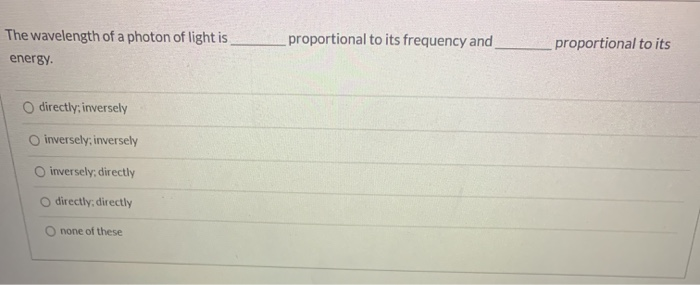The wavelength of a photon of light is _ energy. proportional to its frequency and proportional to its O directly, inversely O inversely, inversely O inversely, directly O directly, directly none of these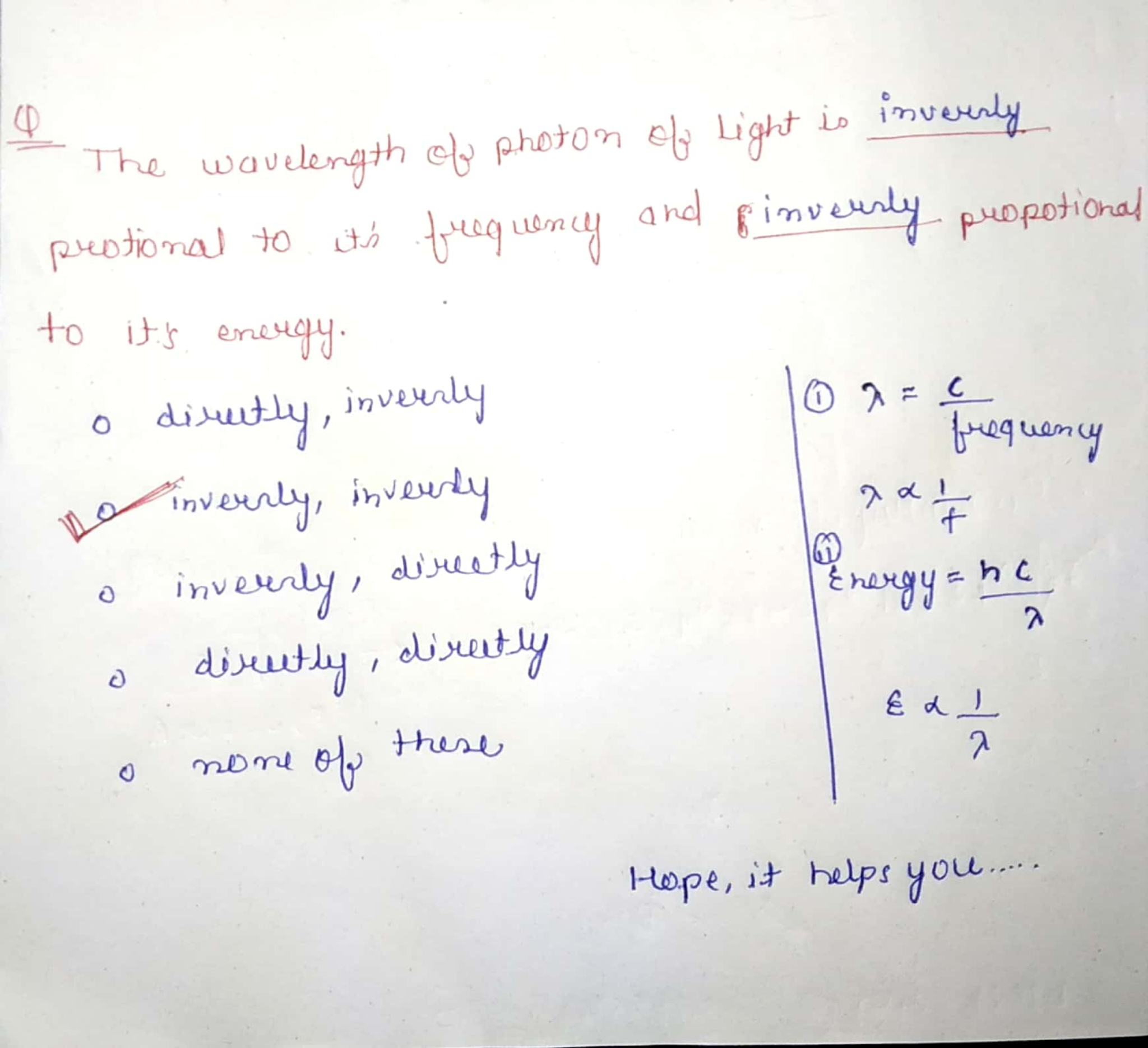#### Earn Coins

Coins can be redeemed for fabulous gifts.

Similar Homework Help Questions
• ### Identify the statement that correctly describes the relationship between wavelength and frequency. Wavelength and frequency are...

Identify the statement that correctly describes the relationship between wavelength and frequency. Wavelength and frequency are directly proportional. Wavelength is independent of frequency. Wavelength and frequency are inversely proportional.   Identify the statement that correctly describes the relationship between wavelength and energy. Energy is independent of wavelength. Energy and wavelength are inversely proportional. Energy and wavelength are directly proportional.   Identify the statement that correctly describes the relationship between energy and frequency. Energy and frequency are inversely proportional. Energy and...

• ### In scenario A, visible light has a wavelength of 700.6 nm. Determine its frequency, energy per...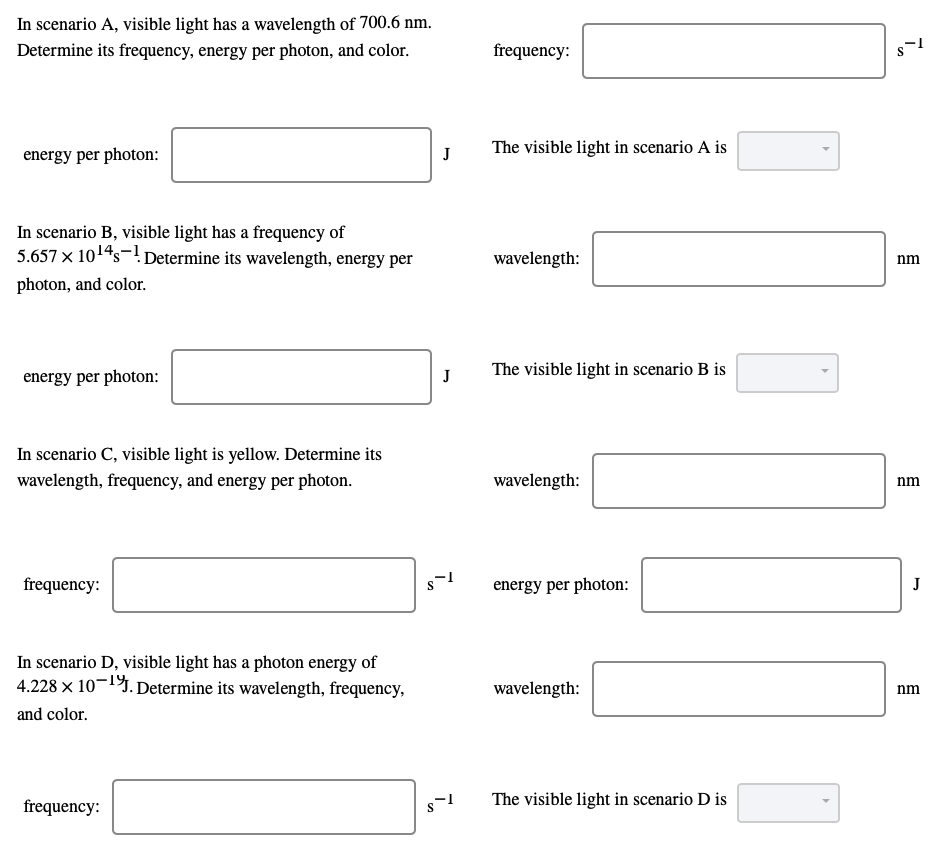In scenario A, visible light has a wavelength of 700.6 nm. Determine its frequency, energy per photon, and color. frequency: s- 1 energy per photon: J The visible light in scenario A is In scenario B, visible light has a frequency of 5.657 x 10145-! Determine its wavelength, energy per photon, and color. wavelength: nm energy per photon: J The visible light in scenario B is In scenario C, visible light is yellow. Determine its wavelength, frequency, and energy per...

• ### wavelength: In scenario B, visible light has a frequency of 5.502 X 10^-!. Determine its wavelength,...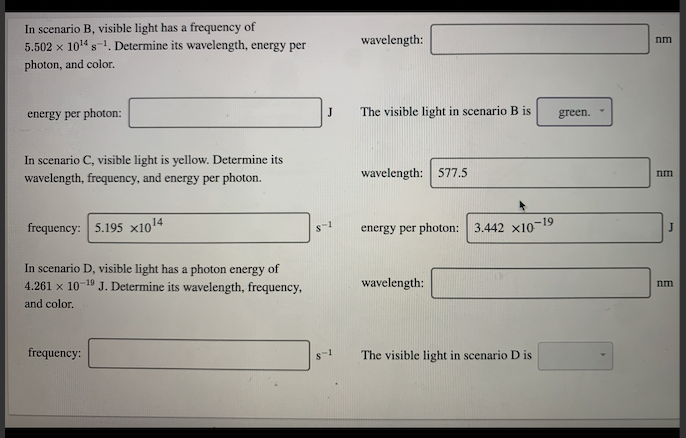wavelength: In scenario B, visible light has a frequency of 5.502 X 10^-!. Determine its wavelength, energy per photon, and color. energy per photon: The visible light in scenario B is green. In scenario C, visible light is yellow. Determine its wavelength, frequency, and energy per photon. wavelength: 577.5 frequency: 5.195 x1014 energy per photon: 3.442 x10-19 In scenario D, visible light has a photon energy of 4.261 x 10-19 J. Determine its wavelength, frequency, and color. wavelength: nm frequency:...

• ### 1. What is the frequency of visible light with a wavelength of 500 nm? 2. For...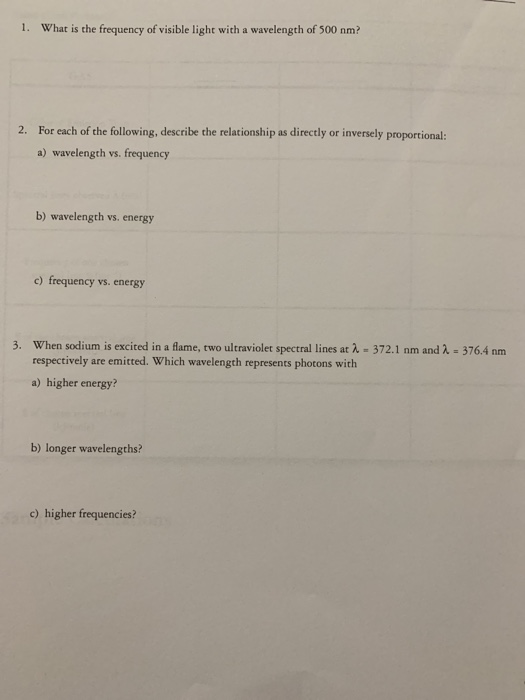1. What is the frequency of visible light with a wavelength of 500 nm? 2. For each of the following, describe the relationship as directly or inversely proportional: a) wavelength vs. frequency b) wavelength vs. energy c) frequency vs. energy -372.1 nm and 2. - 376,4 nm 3. When sodium is excited in a flame, two ultraviolet spectral lines at respectively are emitted. Which wavelength represents photons with a) higher energy? b) longer wavelengths? c) higher frequencies?

• ### 1. Ultraviolet radiation has a frequency of 6.8 x 101 1/3. Calculate the energy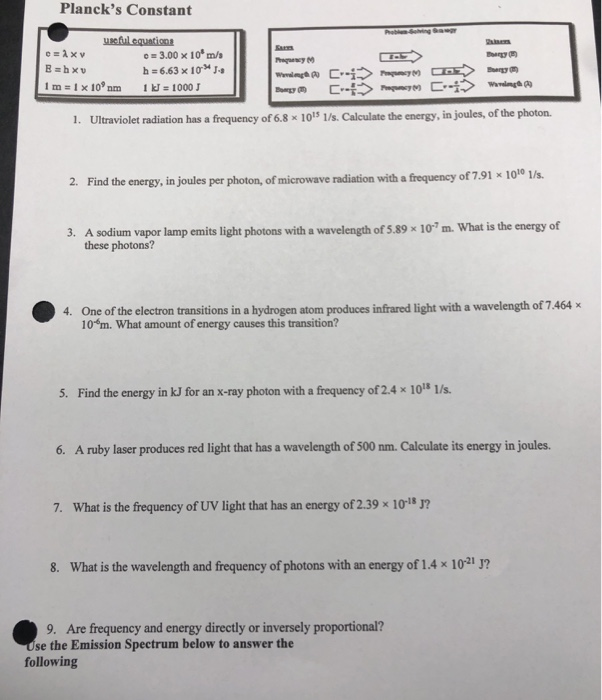.1. Ultraviolet radiation has a frequency of 6.8 x 101 1/3. Calculate the energy, in joules, of the photon. 2. Find the energy, in joules per photon, of microwave radiation with a frequency of 7.91 x 10101/s. 3. A sodium vapor lamp emits light photons with a wavelength of 5.89 x 10m. What is the energy of these photons? 4. One of the electron transitions in a hydrogen atom produces infrared light with a wavelength of 7.464x 10m. What amount of energy causes...

• ### 8- Calculate the energy (E) and wavelength (1) of a photon of light with a frequency...8- Calculate the energy (E) and wavelength (1) of a photon of light with a frequency (v) of 6.16x10²4 Hz. (6 points)

• ### QUESTION 24 What is the relationship between wavelength of light and the quantity of energy per...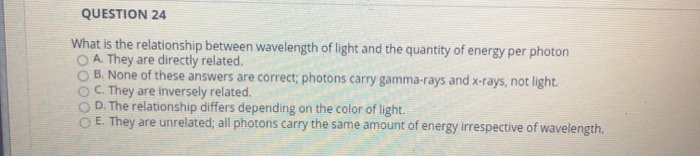QUESTION 24 What is the relationship between wavelength of light and the quantity of energy per photon A. They are directly related. B. None of these answers are correct; photons carry gamma-rays and x-rays, not light. C. They are inversely related. D. The relationship differs depending on the color of light. E. They are unrelated; all photons carry the same amount of energy irrespective of wavelength.

• ### 5 points) What are the frequency. V. and photon ene wavelength of 337.1 nm? and photon energy. E. of light having a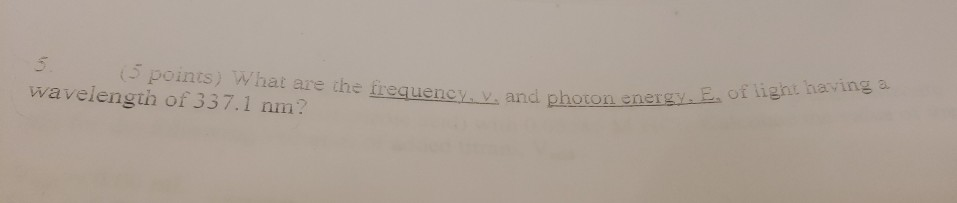5 points) What are the frequency. V. and photon ene wavelength of 337.1 nm? and photon energy. E. of light having a

• ### 2. Calculate the frequency of a photon of green light with a wavelength of 550 nm...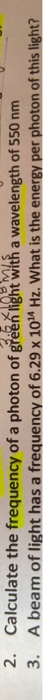2. Calculate the frequency of a photon of green light with a wavelength of 550 nm V 3. A beam of light has a frequency of 6.29 x 104 Hz. What is the energy per photon of this light?

• ### a) What is the wavelength ? in nm for light with a photon energy Ephoton =...

a) What is the wavelength ? in nm for light with a photon energy Ephoton = 2 eV? b) What is the photon energy for light with a wavelength ? = 600 nm? c) What is the photon energy for light with a wavelength ? = 630 nm? (in units of eV)